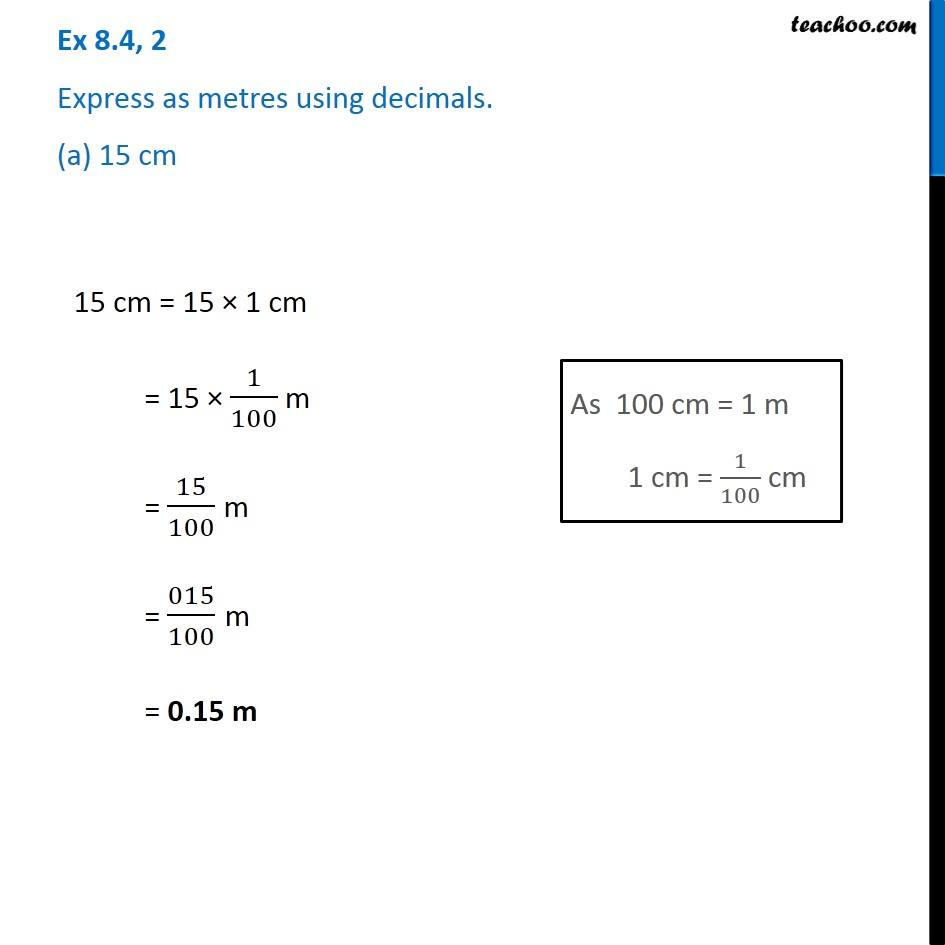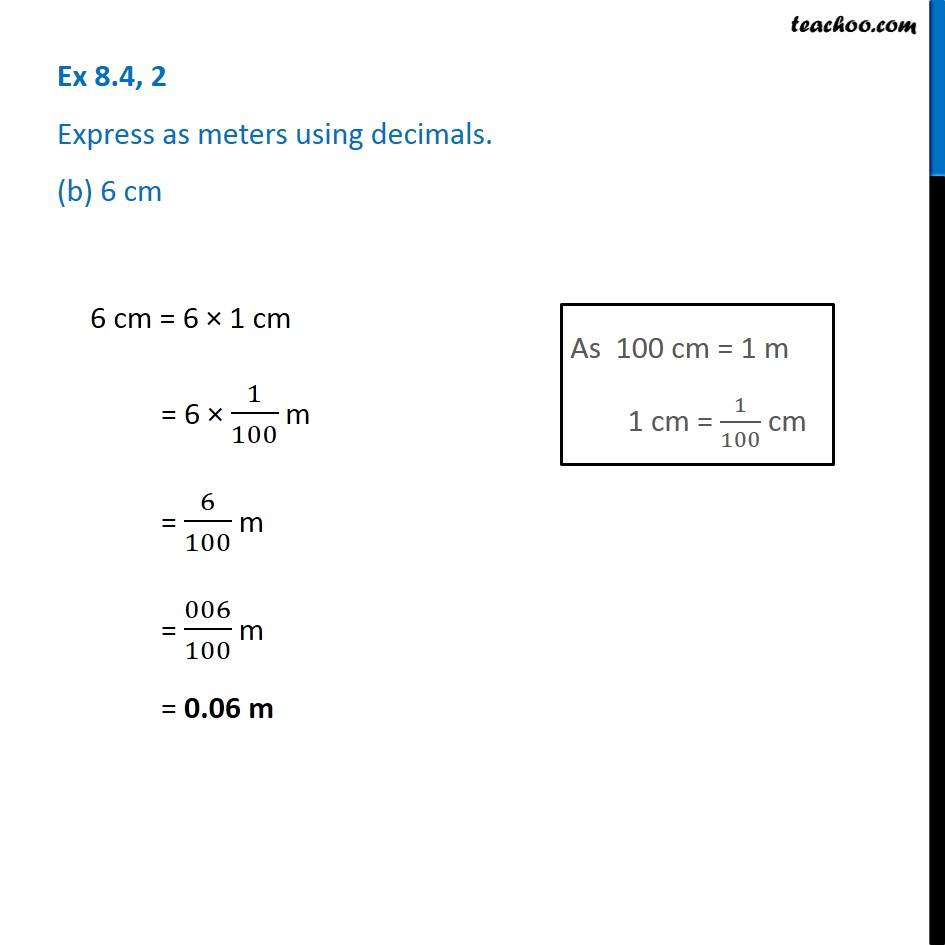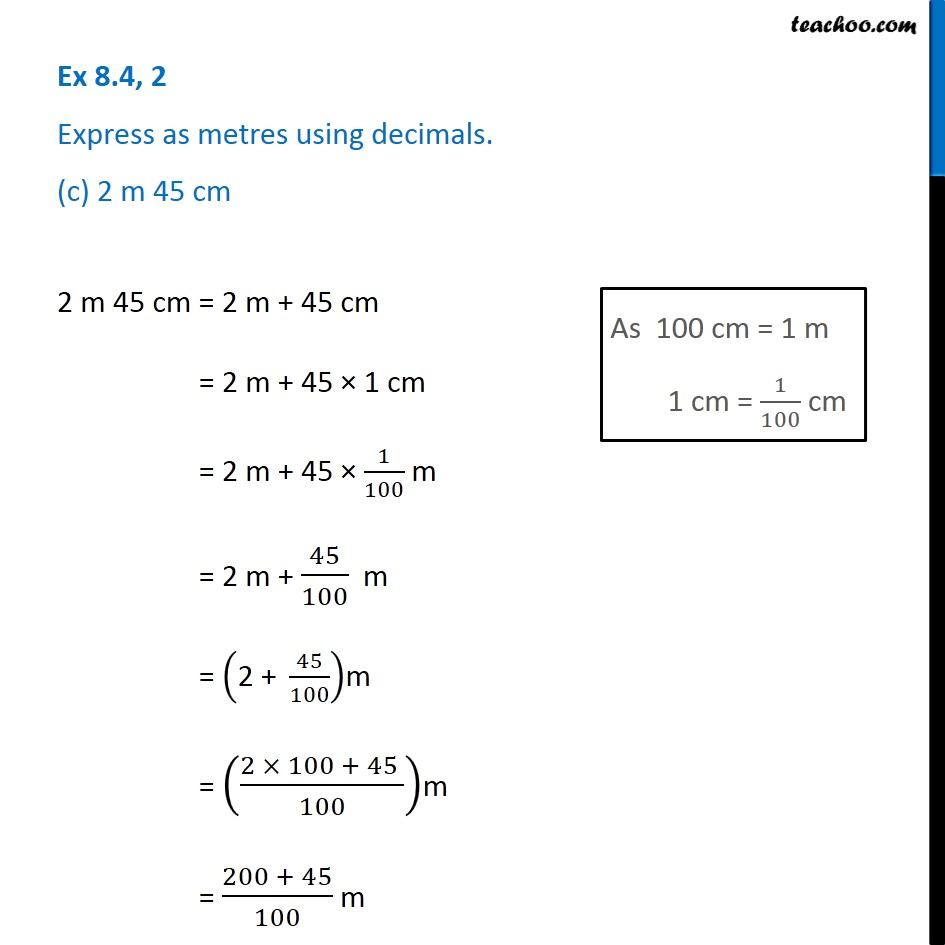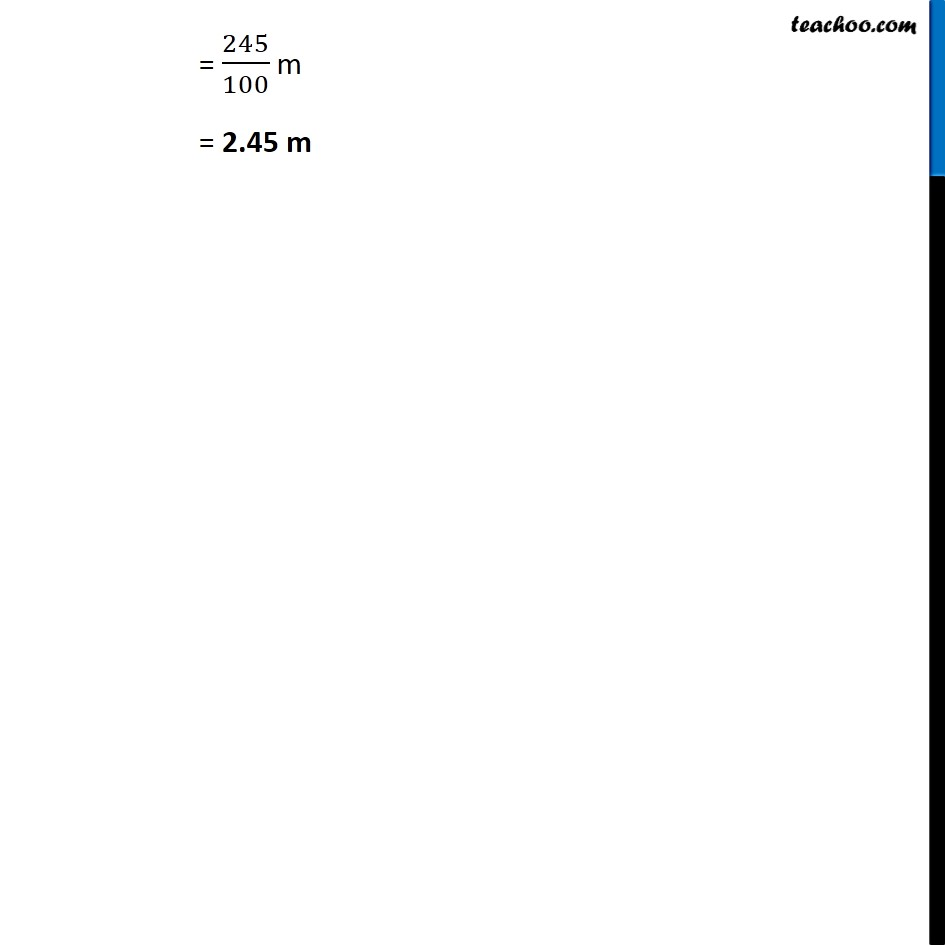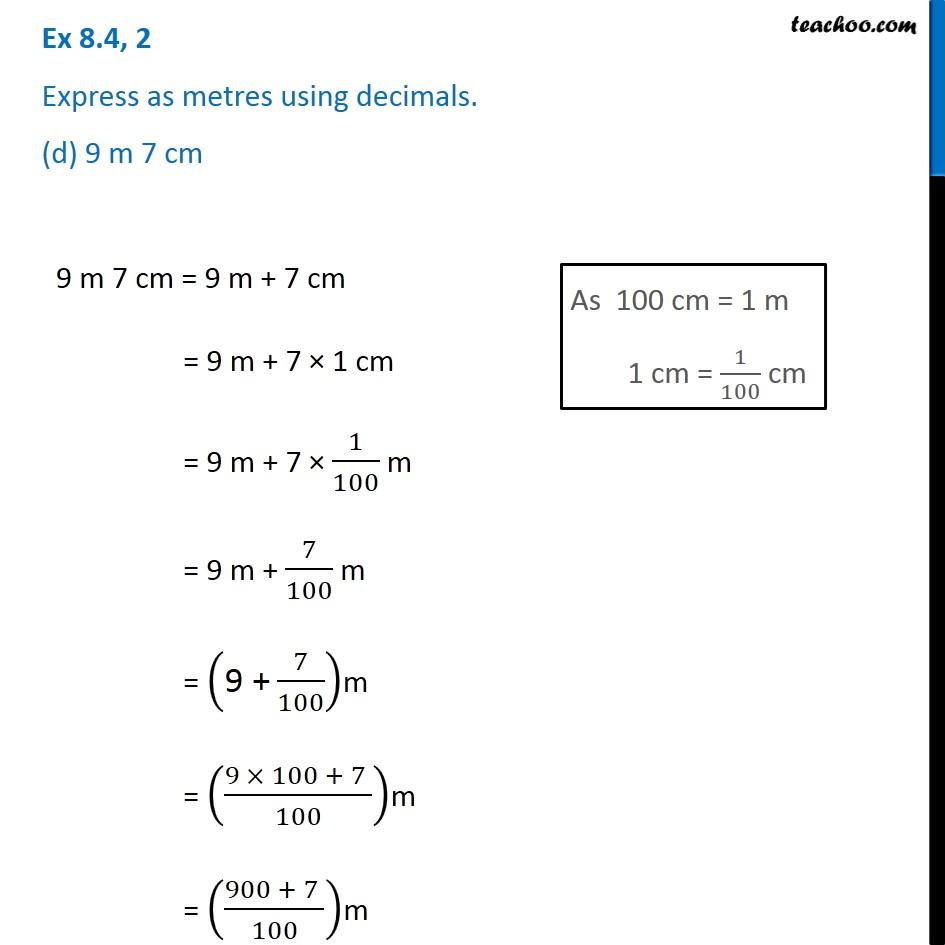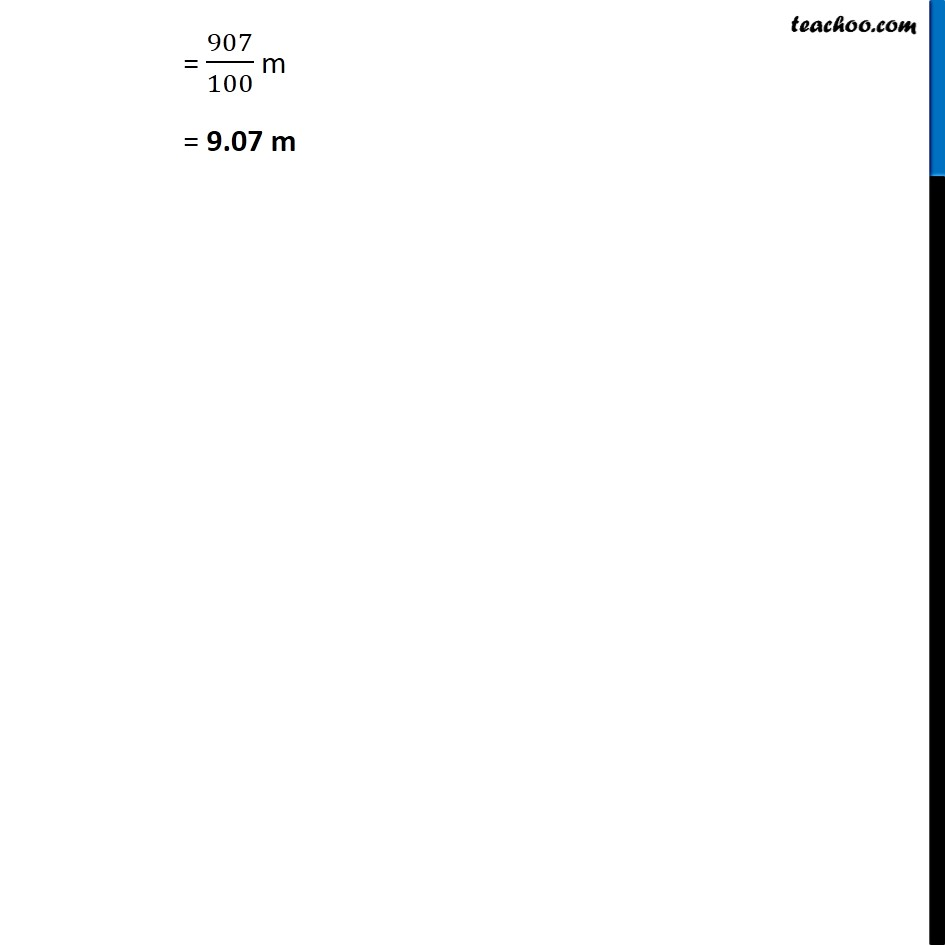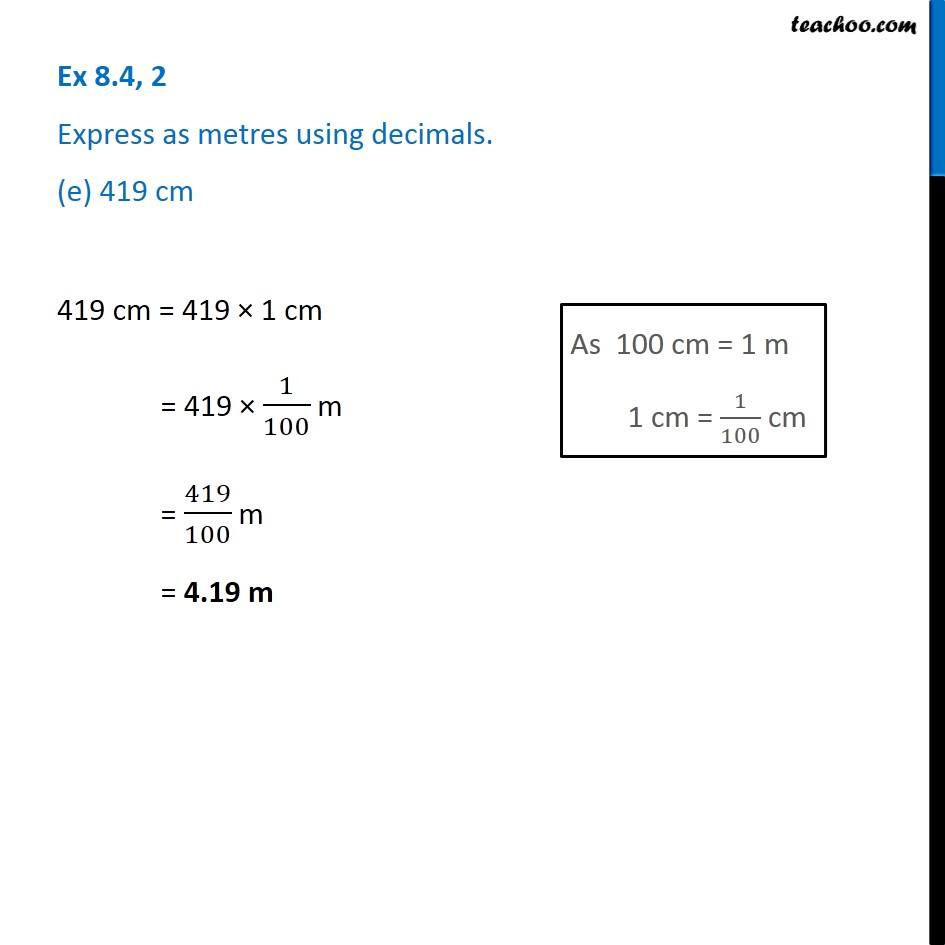1. Chapter 8 Class 6 Decimals
2. Serial order wise
3. Ex 8.4

Transcript

Ex 8.4, 2 Express as metres using decimals. (a) 15 cm 15 cm = 15 × 1 cm = 15 × 1/100 m = 15/100 m = 015/100 m = 0.15 m Ex 8.4, 2 Express as meters using decimals. (b) 6 cm 6 cm = 6 × 1 cm = 6 × 1/100 m = 6/100 m = 006/100 m = 0.06 m Ex 8.4, 2 Express as metres using decimals. (c) 2 m 45 cm 2 m 45 cm = 2 m + 45 cm = 2 m + 45 × 1 cm = 2 m + 45 × 1/100 m = 2 m + 45/100 m = ("2 + " 45/100)m = ((2 × 100 + 45 )/100)m = (200 + 45)/100 m Ex 8.4, 2 Express as metres using decimals. (d) 9 m 7 cm 9 m 7 cm = 9 m + 7 cm = 9 m + 7 × 1 cm = 9 m + 7 × 1/100 m = 9 m + 7/100 m = ("9 +" 7/100)m = ((9 × 100 + 7 )/100)m = ((900 + 7 )/100)m Ex 8.4, 2 Express as metres using decimals. (e) 419 cm 419 cm = 419 × 1 cm = 419 × 1/100 m = 419/100 m = 4.19 m

Ex 8.4

Chapter 8 Class 6 Decimals
Serial order wise